Choose Language Hide Translation Bar
Potato chips and ANOVA, Part 2: Using analysis of variance to improve sample preparation in analytical chemistry

Editor's Note: This post was written by @eric_cai, a chemist and statistician who also blogs at The Chemical Statistician. Follow Eric on Twitter @chemstateric.

In an earlier blog post, I began a two-part series to examine a proposed sample preparation scheme for measuring the weight percentage of sodium in potato chips.  My first post:

• Introduced the problem.
• Entered the raw data into JMP
• Standardized the format of the data using Standardize Attributes.
• Used the Stack function to shape our data into a format that is ready for analysis.

In this second post, I will use the Fit Y by X platform in JMP to visualize the data and analyze them using analysis of variance (ANOVA). I will conclude this series by interpreting the ANOVA results to answer our original question about comparing the variation in the two stages of our sample preparation scheme.

Visualizing the Variation

Our goal is to compare the variation between the two stages of sample preparation. Before performing any statistical analysis, let’s visualize the data using a scatterplot with the grand mean and the group-specific means drawn. Then, we will use ANOVA to answer our question. Fortunately, JMP has one platform that allows you to do both things. Under the Analyze menu, choose Fit Y by X.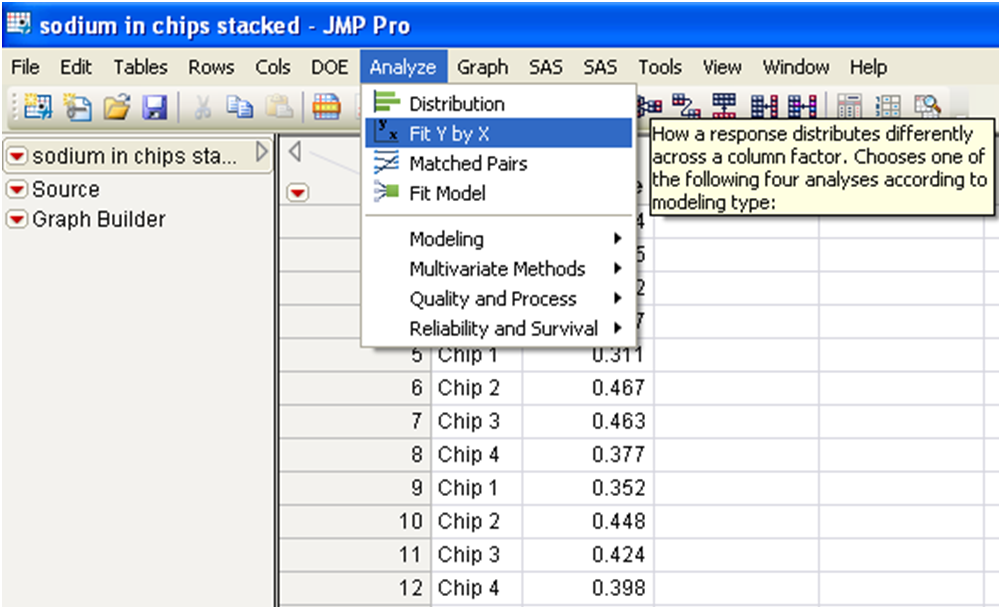In the Fit Y by X platform, choose Weight Percentage as the response (Y), and choose Chip as the factor (X) – view my example below if needed. Notice that JMP automatically recognizes this model as a one-way ANOVA – see the Oneway label appearing in the lower half of the left side of this window. Click OK to run this model.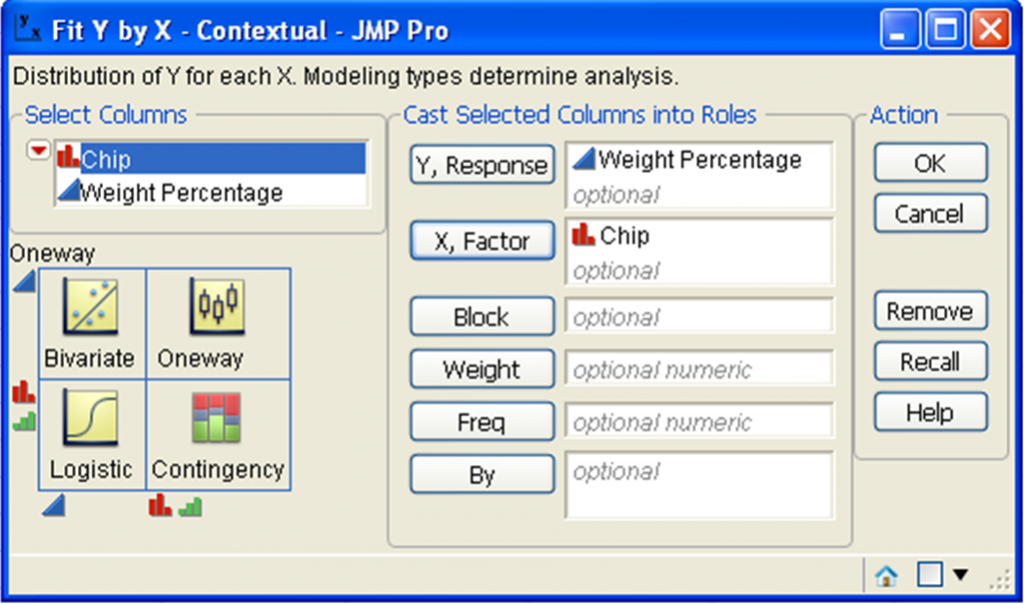The initial output that you get is just a scatterplot of the data. However, there is a lot more information that you can get from the red triangle menu on the upper-left corner. First, let’s enlarge the markers for the points for easier visualization. Right-click anywhere within the plot to adjust the marker size.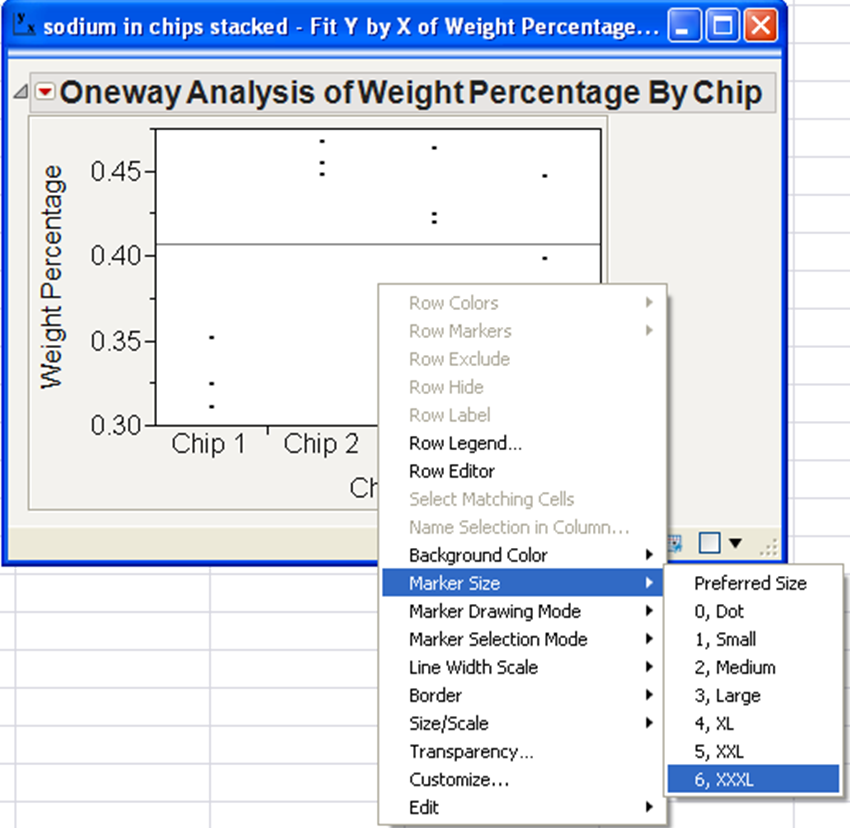For our later statistical analysis, the grand mean (the mean of all weight percentages) and the four group-specific (or chip-specific) means will become important. The grand mean is shown by default. Let’s also visualize the four chip-specific means on this scatterplot. Under the red-triangle menu, go to Display Options, and choose Mean Lines.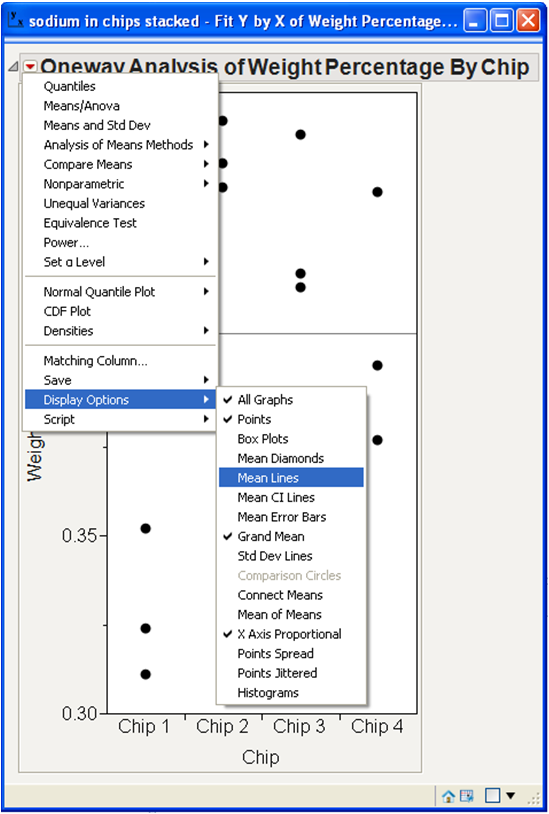The lines for the grand mean and the chip-specific means are too narrow to be seen clearly, so let’s widen them. Right-click anywhere in the plot, and go to the Line Width menu. Let’s choose Other to widen the line to 6.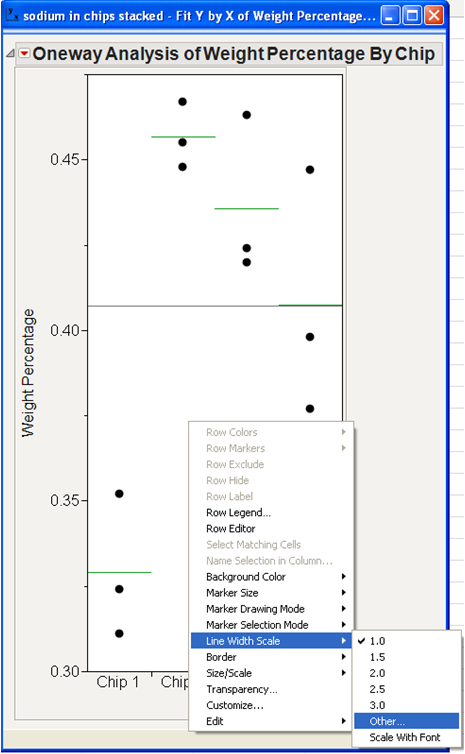Here is the resulting plot with the wider lines.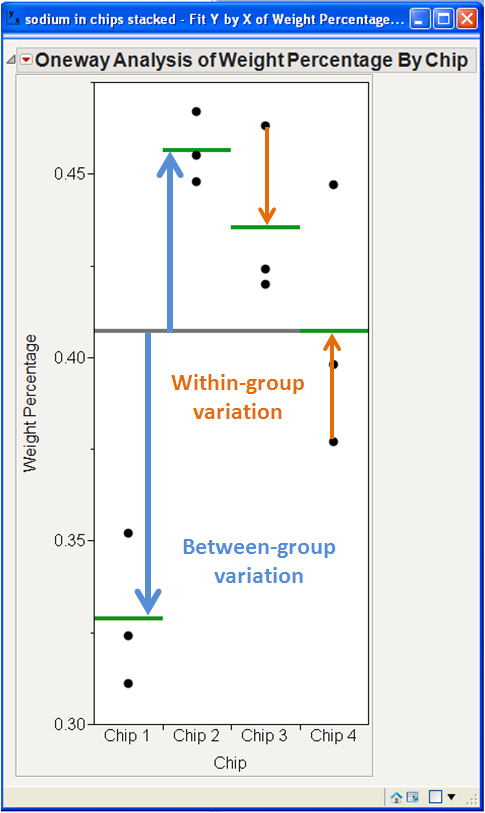Those points and lines are much easier to see. I have manually added some arrows and text in blue and orange using Microsoft Word to partition the two different types of variation that are introduced in the sampling process:

• Drawing four different chips with slightly different weight percentages of sodium from the bag

• Drawing three different aliquots from each flask of the homogenized solution for each chip

In short, there is variation between the four chip samples, and there is variation within each chip sample. The total variation in the final measured values of the weight percentages originate from these two sources of variation. (For the sake of brevity and simplicity, I am ignoring the propagation of measurement uncertainty. If sufficiently good equipment is used, this uncertainty should be minimal compared to the variation introduced by these two steps of sampling.) The key question is this: Which step contributes more to the total variation (and, therefore, the cumulative uncertainty) in the measured weight percentages of sodium?

Partitioning Variation Using ANOVA

A good statistical technique for partitioning and comparing sources of variation is analysis of variance, or ANOVA. It uses the concept of sum of squares to rigorously measure variation – whether it is between groups or within groups. This formulation is applied to both the between-group variation and the within-group variation.

To implement ANOVA, click on Means/ANOVA under the red-triangle menu.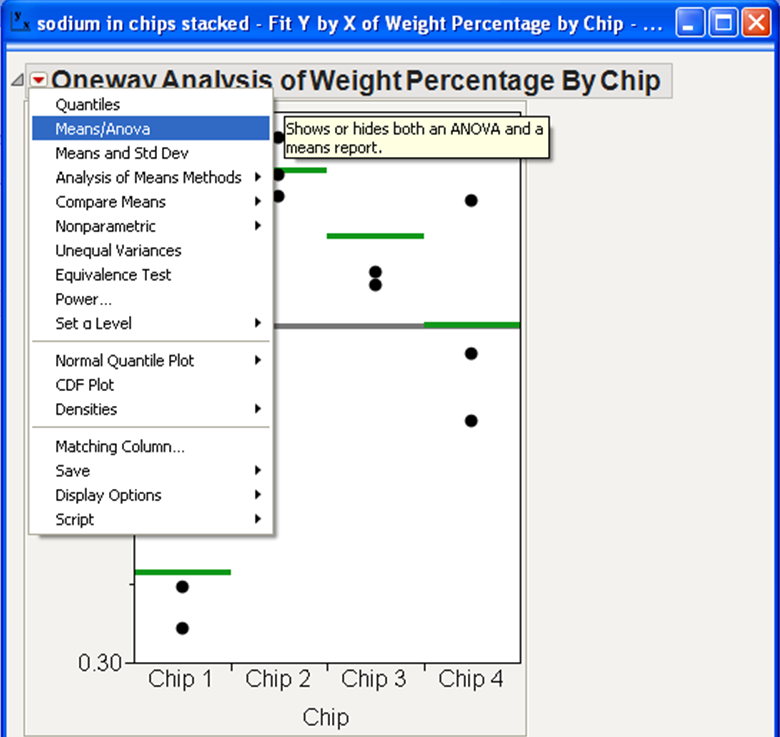The ANOVA report will appear, and the mean diamonds will be added to the scatterplot by default.  I prefer to look at the plot without the mean diamonds, so I clicked on the red-triangle menu, went to Display Options, and unchecked Mean Diamonds. Here is the resulting output: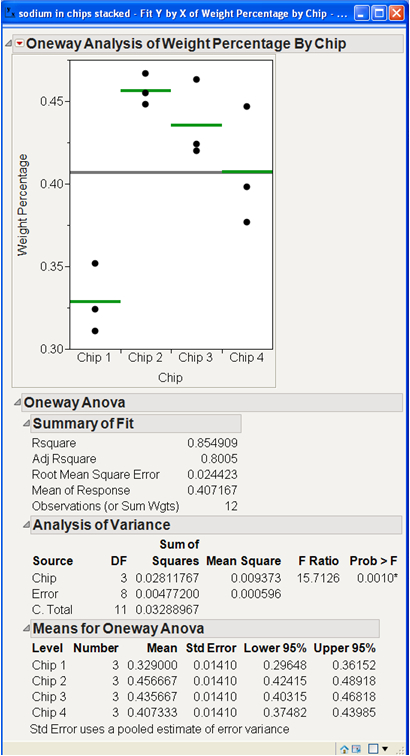Interpreting the Results

We are now ready to use the ANOVA results to answer our original question. Look under Analysis of Variance in the above screenshot. The mean of the sum of squares – abbreviated as Mean Square in the output above, quantifies the variation in each stage of the sampling process.

• The row titled Chip refers to the between-group variation – this is the variation in the weight percentages of sodium between the four chips that were originally drawn from the bag.

• The row titled Error refers to the within-group variation – this is the variation in the weight percentages of sodium between the aliquots within each chip. It combines this variation from all four chips.

Notice that the between-group variation is much higher than the within-group variation – 0.009373 compared to 0.000596. Under the null hypothesis that the two sources of variation are equal, the ratio of these two mean squares has an F-distribution. You can test whether or not this ratio is significantly bigger than 1. (An F ratio of 1 implies that the two sources of variation are equal.) The P-value of that test (Prob > F) is 0.0010, suggesting that creating four separate homogenized solutions produced much more variation than drawing the 12 aliquots.

Revising the Sample Preparation Process

We have shown that our proposed sample preparation process contributes far more variation in the first stage of sampling than in the second stage. This prompts thinking of a new way to sample the chips before drawing aliquots from their homogenized solutions for measurement.

Here is an alternative sample preparation scheme that reduces the initial sampling variation.

• Draw the four chips from the bag.

• Blend the four chips together and homogenize them into one solution.

• Draw aliquots from this one solution.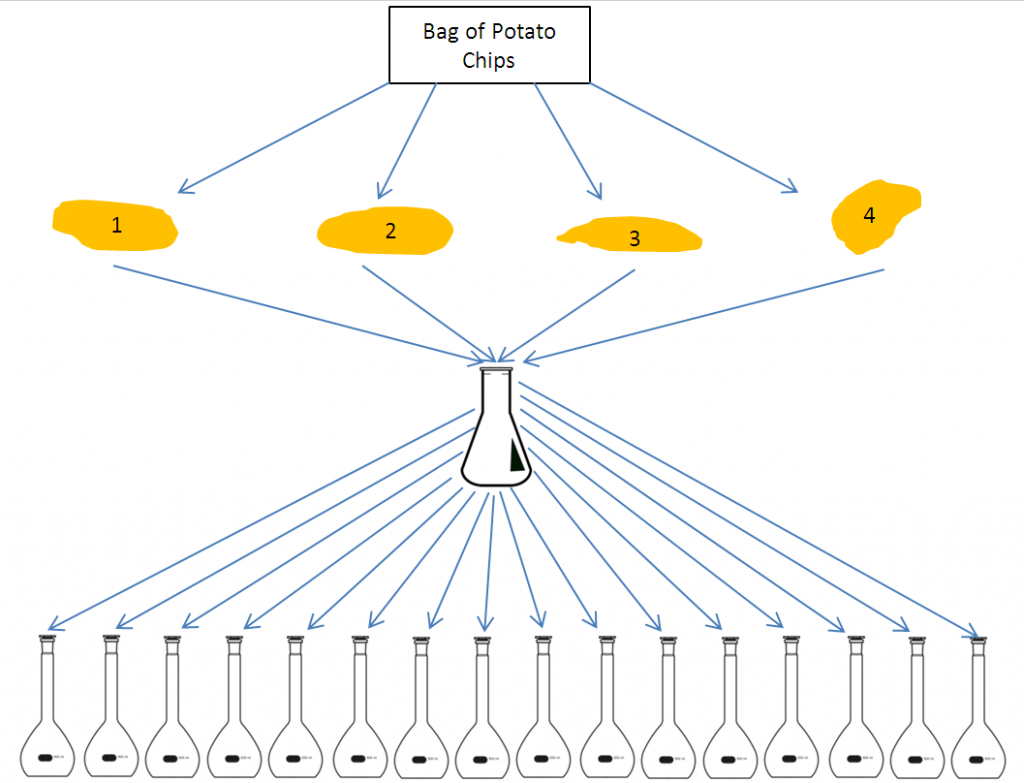A rigorous mathematical derivation can show that the total variance in this alternative scheme is smaller than the total variance in the first scheme proposed at the beginning of this blog post. For the sake of brevity, I will not show this derivation in this blog post, but you can find a sketch of this derivation on pages 75-78 in Chapter 4 in Miller and Miller (2010).

However, there is a disadvantage to this blending strategy – more aliquots need to be drawn and analyzed. These additional measurements cost more time, equipment or money, so this blending strategy is not always the best method. Past experiences can inform you on the between-group and within-group variances and the costs of additional sampling and measurement. A careful balance between cost and precision can help you to achieve the most cost-effective way to answer your analytical question.

References

Harris, D. C. (2002). Quantitative chemical analysis (6th edition). Macmillan.

Miller, J. N., & Miller, J. C. (2010). Statistics and chemometrics for analytical chemistry (6th edition). Pearson Education.

Article Labels

There are no labels assigned to this post.